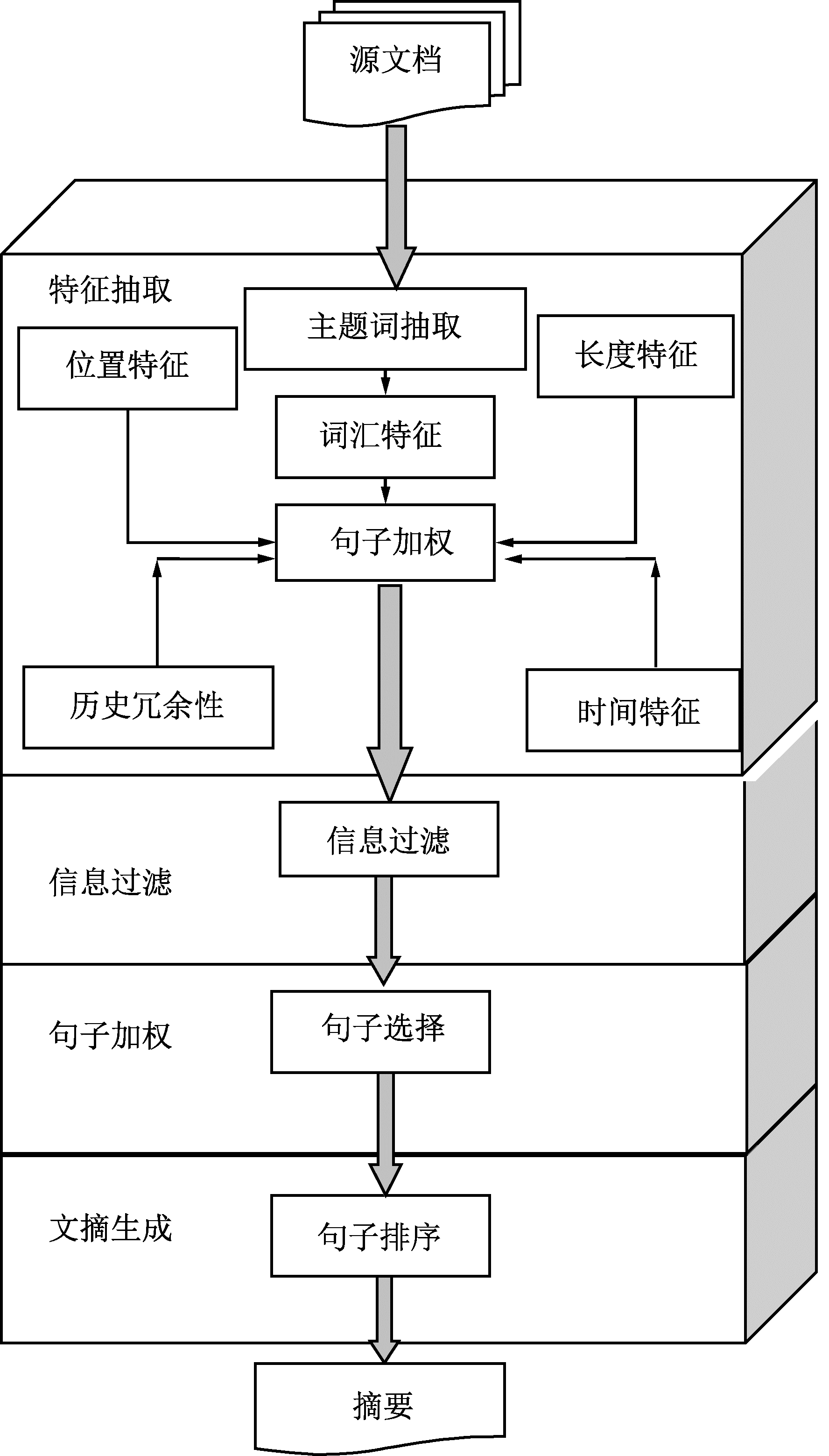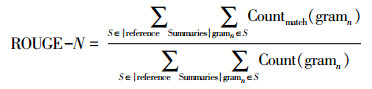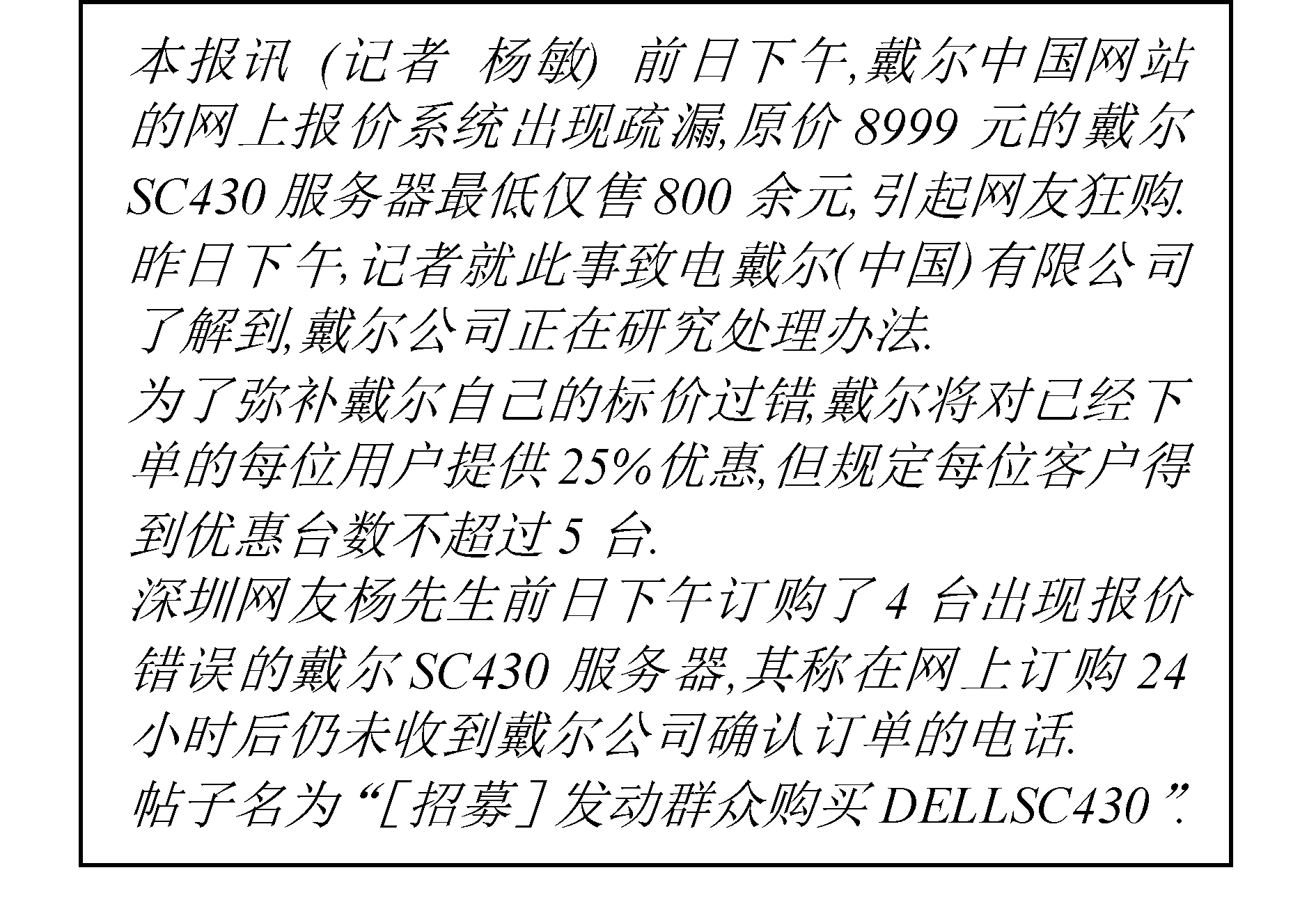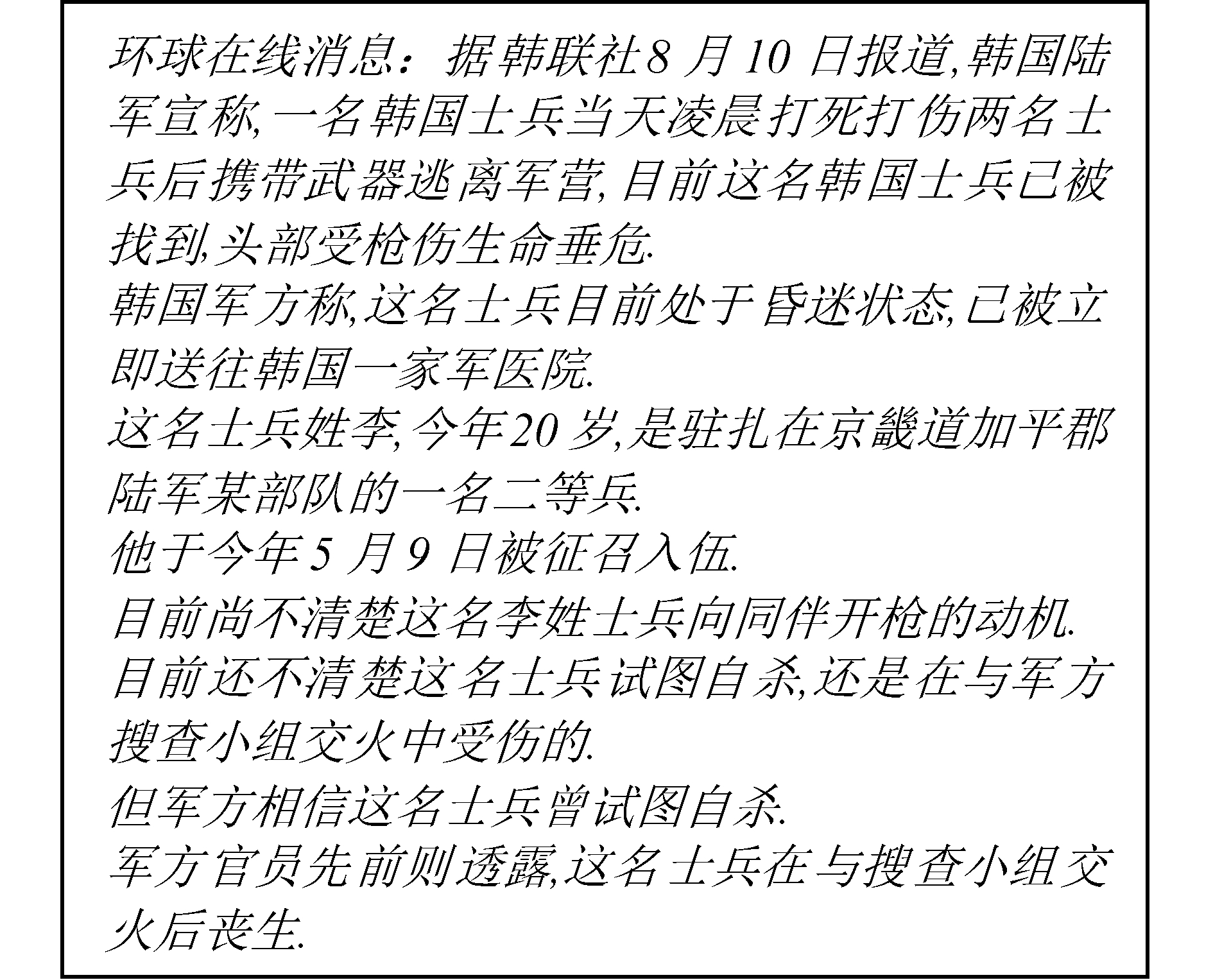﻿ 中文文本挖掘的动态文摘建模方法
«上一篇文章快速检索 高级检索

 哈尔滨工程大学学报2019, Vol. 40Issue (4): 820-826  DOI: 10.11990/jheu.2017100620

### 引用本文LIU Meiling, WANG Huiqiang, CHEN Guangsheng, et al. Research dynamic summarization modeling method based on Chinese text mining[J]. Journal of Harbin Engineering University, 2019, 40(4), 820-826. DOI: 10.11990/jheu.201710062.### 文章历史

1. 东北林业大学 信息与计算机工程学院, 黑龙江 哈尔滨 150040;
2. 哈尔滨工程大学 计算机科学与技术学院, 黑龙江 哈尔滨 150001

Research dynamic summarization modeling method based on Chinese text mining
LIU Meiling 1,2, WANG Huiqiang 2, CHEN Guangsheng 1, YU Yang 1
1. College of Information and Computer Engineering, Northeast Forestry University, Harbin 150040, China;
2. College of Computer Science and Technology, Harbin Engineering University, Harbin 150001, China
Abstract: Chinese summarization is one of the most important research fields in natural language processing.In particular, the study of time characteristics in comprehension-based abstracts has attracted widespread attention.In this study, we extract the dynamic features of Chinese text summary and conduct text mining and modeling analysis to reflect the time characteristics.Based on dynamic performance, a dynamic summarization model is established and a Chinese summarization evaluation method is proposed by constructing the framework of Chinese dynamic multi-document summarization system and the algorithm design of key steps, including sentence weighting, feature extraction, and sentence selection.Experiments show that the dynamic Chinese summarization technology and its evaluation algorithm can obtain understandable time-lapse summaries in the actual text mining process, which is feasible and possesses high research value.
Keywords: dynamic abstract    modeling    text mining    Chinese abstract    evaluation method    sentence weighting    text understanding    corpus testing

1 研究现状分析 1.1 中文多文档文摘及动态文摘发展现状

1.2 中文多文档文摘系统的自动评测

BLEU是一种经典评测工具。它以精确率为主要研究目标，对基于原文抽取的自动文摘进行评价，也被大量运用在机器翻译当中。ROUGE(recall-oriented understudy for gisting evaluation)[14-15]是一种重要的评价标准，在国际知名评测中被采用作为主要的评价方法。ROUGE-N计算系统生成文摘与人工文摘之间同现单元的个数，是一个用来评价的态演化性的指标。

2 CDMDS建模方法Download: 图 1 CDMDS框架 Fig. 1 CDMDS framework
2.1 特征抽取模块 2.1.1 主题词抽取及其权值计算

1) 主题签名的定义。

 $\begin{array}{l} \;\;\;\;\;\;{T_S} = \left\{ {{\rm{topic}}, {\rm{signature}}} \right\} = \\ \;\left\{ {{\rm{topic}}, \left\langle {\left( {{t_1}, {w_1}} \right), \cdots , \left( {{t_n}, {w_n}} \right)} \right\rangle } \right\} \end{array}$ (1)

2) 基于同现统计的主题签名自动获取。

2.1.2 句子历史冗余性特征值的计算

 ${N_{{\rm{Wgt}}}}\left( s \right) = \left\{ {\sum\limits_{i = 1}^m {\left( {\frac{{\sum\limits_{j = 1}^n {{W_{{\rm{gt}}}}\left( {{w_{_{\rm{j}}}}} \right)} }}{{{\rm{length}}\left( {{s_i}} \right)}}} \right)} } \right\}/{\rm{length}}\left( s \right) \cdot {\rm{count}}$ (2)

2.1.3 句子显著性特征值的计算

 ${S_{{\rm{Wgt}}}}\left( s \right) = \left\{ {\sum\limits_{i = 1}^m {\left( {\frac{{\sum\limits_{j = 1}^n {{\rm{Weight}}\left( {{w_{_\mathit{j}}}} \right)} }}{{{\rm{length}}\left( {{s_i}} \right)}}} \right)} } \right\}/{\rm{length}}\left( s \right) \cdot {\rm{count}}$ (3)

2.1.4 句子时间特征值的计算

 ${T_{{\rm{Wgt}}}}\left( s \right) = 1/n$ (4)

2.1.5 句子长度特征值的计算

 $\begin{array}{l} \;\;\;\;\;{L_{{\rm{Wgt}}}}\left( s \right) = 1/\left( {{\rm{Length}}\left( s \right) - 0.5 \cdot } \right.\\ \left. {{\rm{MaxLength}}} \right){\rm{Length}}\left( s \right) > 0.5 * {\rm{MaxLength}} \end{array}$ (5)

2.1.6 句子位置特征权值的计算

 ${P_{{\rm{Wgt}}}}\left( s \right) = 1/n$ (6)

2.2 信息过滤模块

2.3 句子加权模块

2.4 候选句生成模块

 $\begin{array}{l} \;\;\;\;\;\;\;\;\;\;\;\;\;\;{A_{{\rm{ZWgt}}}}\left( s \right) = \\ {\rm{ }}\alpha \cdot \left( {{B_{{\rm{ZWgt}}}}\left( s \right) - \beta \cdot \sum\limits_{i = 1}^n {\frac{{\sum\limits_{j = 1}^{{\rm{simcount}}} {{{\rm{W}}_{{\rm{gt}}}}\left( {{w_j}} \right)} }}{{\sum\limits_{k = 1}^{{\rm{count}}\left( {{s_i}} \right)} {{W_{{\rm{gt}}}}\left( {{w_k}} \right)} }}} } \right) \end{array}$ (7)

3 中文动态多文档文摘评测方法 3.1 ROUGE评测方法(8)

2-gram文法结构即依次连续抽取2个单词，以此可得出n-gram的定义。参考句中共有6个词，参考句与之相同的词有1个，因此2-gram匹配度为1/6。

3.2 中文评测指标

1) CROUGE-1评测指标。

 ${\rm{CROUGE}} - 1 = \frac{{\sum {{\rm{Coun}}{{\rm{t}}_{{\rm{match}}}}\left( {{\rm{Unigram}}} \right)} }}{{\sum {{\rm{Count}}\left( {{\rm{Unigram}}} \right)} }}$ (9)

2) CROUGE-2评测指标。

CROUGE-1虽然能够估计出系统文摘与参考文摘之间的一元词语匹配率，但是这不足以说明其相互之间的内容匹配率，以及其内容之间的相似度。例如“他打了别人”和“别人打了他”，这两句话的一元词语匹配率达到了百分之百，但是两句话的意思是截然不同的，若用CROUGE-1指标进行评测，其性能为佳。这就足以说明ROUGE-1指标单用时效果不佳，为了克服该指标单用时的缺点，本评测系统又从英文评测系统中引进了ROUGE-2评测指标，即为CROUGE-2。

CROUGE-2对中文动态文摘系统而言是另一重要评测指标，是为了更好地评测中文文摘系统的性能而引进的又一评测指标，其克服了CROUGE-1单独使用时的缺点，CROUGE-2以相连的2个汉字为研究对象，计算系统文摘对参考文摘中相连双汉字的覆盖率，此指标跟CROUGE-1合用时能够准确地估计出系统文摘对参考文摘内容的覆盖度，进而对文摘系统的性能进行高效率的估计，其计算公式为：

 ${\rm{CROUGE}} - 2 = \frac{{\sum {{\rm{Coun}}{{\rm{t}}_{{\rm{match}}}}\left( {{\rm{Bigram}}} \right)} }}{{\sum {{\rm{Count}}\left( {{\rm{Bigram}}} \right)} }}$ (10)

3) CROUGE-SU4评测指标。

CROUGE-2统计的数据为参考文摘与系统文摘中二元语法同现率，虽然能够在一定程度上评价文摘系统的性能，但是不够全面。因为在中文文章中还存在另一种语言现象，即固定搭配，语言中的固定搭配现象很多，本文研究最具代表性的一种固定搭配现象，即二元固定搭配，此类固定搭配中只有2个中文字，但是此二字之间可以包含任意数量的字。本文对二字之间具有四字的二元固定搭配进行研究，也即英文评测系统ROUGE评测工具中的ROUGE-SU4评测指标，记为CROUGE-SU4，其能很好地克服CROUGE-1和CROUGE-2评测指标的不足，从另外一个角度对文摘系统的性能进行评测。其评测结果也更全面更具说服力。其计算公式为：

 ${\rm{CROUGE}} - {\rm{SU}}4 = \frac{{\sum {{\rm{Coun}}{{\rm{t}}_{{\rm{match}}}}\left( {{\rm{skip\_four\_gram}}} \right)} }}{{\sum {{\rm{Count}}\left( {{\rm{skip\_four\_gram}}} \right)} }}$ (11)

4 实验结果与分析 4.1 实验语料库介绍表 1 中文测试语料库 Table 1 Table of Chinese test corpus
4.2 中文动态文摘结果Download: 图 2 ‘戴尔网络报价出错′主题文档集文摘结果 Fig. 2 "DELL network quotes error" subject document collection summary resultsDownload: 图 3 ‘韩国士兵枪杀同伴后携武器出逃′文摘结果 Fig. 3 "South Korean soldiers shot companions carrying weapons fled" abstract results
4.3 评测结果及分析表 2 中文系统评测结果 Table 2 evaluation results of Chinese system

5 结论

1) 通过话题检测与跟踪技术(TDT)与多文档文摘技术的结合，更深刻地理解时序特征对信息处理技术的重要性，得出了相应结论；

2) 对于基于网络动态数据的信息处理领域，动态多文档文摘技术和TDT技术都与动态演化性密切相关。通过对中文特点的分析，将动态多文档文摘模型应用到中文领域进行研究实现了中文的动态多文档文摘系统；

3) 本文开发了类似于英文评测标准的中文文摘评测系统，目前中文领域缺乏的就是统一的标准的评测机制，很多学者也做了这方面的研究, 但是缺乏权威性，这和中文特殊的语言环境有关。本文的评测系统仿照英文标准的ROUSE评测设置指标，并基于动态数据领域，描述其动态演化性，根据中文特点进行评价。实验表明，此方法有一定的可行性，是一项有价值的研究。

  郭庆琳, 樊孝忠, 柳长安. 基于文本聚类的自动文摘系统的研究与实现[J]. 计算机工程, 2006, 32(4): 30-32, 121. GUO Qinglin, FAN Xiaozhong, LIU Changan. Research and implementation about automatic abstract system based on text clustering[J]. Computer engineering, 2006, 32(4): 30-32, 121. (0)  郭庆琳, 樊孝忠, 柳长安. 文本聚类在自动文摘中的应用研究[J]. 计算机应用, 2005, 25(5): 1036-1038. GUO Qinglin, FAN Xiaozhong, LIU Changan. Application in automatic abstracting for text clustering[J]. Computer application, 2005, 25(5): 1036-1038. (0)  张其文, 李明. 多文档文摘提取方法的研究[J]. 兰州理工大学学报, 2007, 33(1): 96-99. ZHANG Qiwen, LI Ming. Investigation of method for extracting multi-document abstracts[J]. Journal of Lanzhou university of technology, 2007, 33(1): 96-99. DOI:10.3969/j.issn.1673-5196.2007.01.025 (0)  刘美玲, 任洪娥, 于洋, 等. 基于网络的动态多文档文摘系统框架[J]. 软件学报, 2013, 24(5): 1006-1021. LIU Meiling, REN Honge, YU Yang, et al. Web-based dynamic multi-document summarization system framework[J]. Journal of software, 2013, 24(5): 1006-1021. (0)  刘德荣, 王永成, 刘传汉. 基于主题概念的多文档自动摘要研究[J]. 情报学报, 2005, 24(1): 69-74. LIU Derong, WANG Yongcheng, LIU Chuanhan. Study of multiple documents summarization based on subject concept cohesion[J]. Journal of the China society for scientific and technical information, 2005, 24(1): 69-74. DOI:10.3969/j.issn.1000-0135.2005.01.010 (0)  YE Na, ZHU Jingbo, ZHENG Yan, et al. A dynamic programming model for text segmentation based on min-max similarity[C]//Proceedings of the 4th Asia Information Retrieval Conference on Information Retrieval Technology. Harbin, China, 2008: 141-152. (0)  杨选选, 张蕾. 基于语义角色和概念图的信息抽取模型[J]. 计算机应用, 2010, 30(2): 411-414. YANG Xuanxuan, ZHANG Lei. Information extraction based on semantic role and concept graph[J]. Journal of computer applications, 2010, 30(2): 411-414. (0)  张瑾, 许洪波, 程学旗. 面向网络演化信息的动态文摘方法研究[J]. 计算机学报, 2008, 31(4): 696-701. ZHANG Jin, XU Hongbo, CHENG Xueqi. Research on dynamic summarization for evolutionary web information[J]. Chinese journal of computers, 2008, 31(4): 696-701. DOI:10.3321/j.issn:0254-4164.2008.04.015 (0)  ZHANG Jin, CHENG Xueqi, XU Hongbo. Dynamic summarization: Another stride towards summarization[C]//Proceedings of 2007 IEEE/WIC/ACM International Conferences on Web Intelligence and Intelligent Agent Technology-Workshops. Silicon Valley, CA, USA, 2007: 64-67. (0)  余珊珊, 苏锦钿, 李鹏飞. 基于改进的TextRank的自动摘要提取方法[J]. 计算机科学, 2016, 43(6): 240-247. YU Shanshan, SU Jindian, LI Pengfei. Improved TextRank-based method for automatic summarization[J]. Computer science, 2016, 43(6): 240-247. (0)  傅间莲, 陈群秀. 一种新的自动文摘系统评价方法[J]. 计算机工程与应用, 2006, 42(18): 176-177. FU Jianlian, CHEN Qunxiu. A new evaluation method for automatic text summarization[J]. Computer engineering and applications, 2006, 42(18): 176-177. DOI:10.3321/j.issn:1002-8331.2006.18.056 (0)  魏继增, 孙济洲, 秦兵. 多文档文摘评价标准的研究[J]. 计算机工程与应用, 2007, 43(2): 180-183. WEI Jizeng, SUN Jizhou, QIN Bing. Research on standard of evaluation of multi-document summarization[J]. Computer engineering and applications, 2007, 43(2): 180-183. DOI:10.3321/j.issn:1002-8331.2007.02.053 (0)  RUSH A M, CHOPRA S, WESTON J. A neural attention model for abstractive sentence summarization[J]. arXiv preprint arXiv: 1509.00685, 2015. https://www.researchgate.net/publication/281487270_A_Neural_Attention_Model_for_Abstractive_Sentence_Summarization (0)  LIN C Y. Looking for a few good metrics: ROUGE and its evaluation[C]//Proceedings of the NTCIR Workshop. Tokyo, Japan, 2004. (0)  BOUDIN F, MORENO J M T. NEO-CORTEX: a performant user-oriented multi-document summarization system[M]//GELBUKH A. Computational Linguistics and Intelligent Text Processing. Berlin Heidelberg: Springer, 2007: 551-562. (0)  刘美玲, 郑德权, 赵铁军, 等. 动态多文档文摘模型[J]. 软件学报, 2012, 23(2): 289-298. LIU Meiling, ZHENG Dequan, ZHAO Tiejun, et al. Dynamic multi-document summarization model[J]. Journal of software, 2012, 23(2): 289-298. (0)